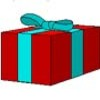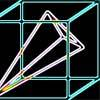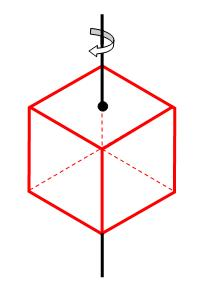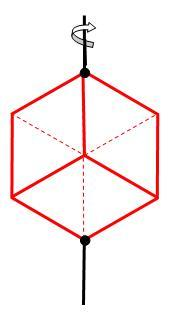#### You may also likeA box of size a cm by b cm by c cm is to be wrapped with a square piece of wrapping paper. Without cutting the paper what is the smallest square this can be?### Plane to See

P is the midpoint of an edge of a cube and Q divides another edge in the ratio 1 to 4. Find the ratio of the volumes of the two pieces of the cube cut by a plane through PQ and a vertex.### Four Points on a Cube

What is the surface area of the tetrahedron with one vertex at O the vertex of a unit cube and the other vertices at the centres of the faces of the cube not containing O?

# Interpenetrating Solids

##### Age 16 to 18Challenge Level

This problem involves visualisation and rotation of cubes. A significant and important part of any visualisation problem is understanding clearly what the problem is asking of you and then thinking about the key features of the problem, so please be prepared to spend some time thinking about what the problem is about before putting pen to paper. Don't forget that sketches, diagrams and models are to be encouraged as they will help you as you work towards the solution .

Imagine that you place a cube on a flat table. You rotate the cube 45 degrees about the axis joining the centre of the top face to the centre of the bottom face.Imagine that the original and rotated cube are superimposed. Imagine also the the resulting shape was sliced along each of the original and rotated faces. How many pieces would the shape fall into and what shapes would they be? Describe the shapes as accurately as possible.

How many rotations give a result where the original and rotated cube are in exactly the same location?Now visualise the same process except with rotations of the cube about an axis passing through directly opposite corners.

A light is shone directly down from above the intersecting cubes. What shadow does it make on the table? This is called making a projection of the cubes.

Finally, suppose that the cube is rotated by 60 degrees about this axis through opposite corners and the original and rotated cube superimposed. The shape is sliced up along the six faces of the original cube What shape are the pieces which would be cut off, and how many are there? If possible give the dimensions of the shapes exactly.

Extension: You may like to consider the problem of rotating other solids such as tetrahedrons and octahedrons.